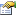﻿ CodecsVectorImageInfo Class Members | Leadtools.Codecs | Raster, Medical, Document Help
←Select platform
In This Topic ▼

CodecsVectorImageInfo Class Members

Summary

The following tables list the members exposed by CodecsVectorImageInfo.

Public Properties

Name DescriptionIsVectorFile Gets a value indicating whether the image file or stream is a vector image format.ParallelogramMaxX The maximum point of the bounding parallelogram of the vector fileParallelogramMaxY The maximum point of the bounding parallelogram of the vector fileParallelogramMaxZ The maximum point of the bounding parallelogram of the vector fileParallelogramMinX The minimum point of the bounding parallelogram of the vector fileParallelogramMinY The minimum point of the bounding parallelogram of the vector fileParallelogramMinZ The minimum point of the bounding parallelogram of the vector fileUnit Gets the unit of measure for the vector image file.